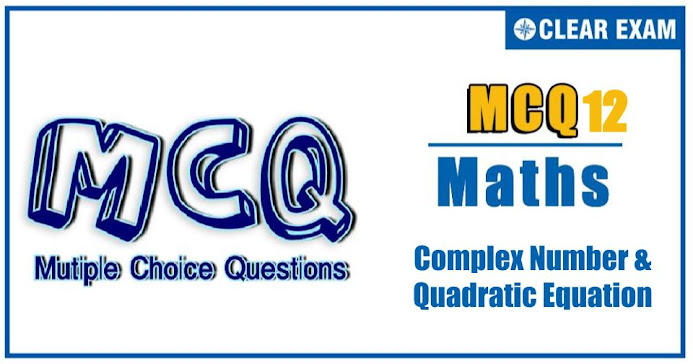## Complex Number & Quadratic Equation Quiz-12

Complex numbers and quadratic equations is a segment of maths that deals with crucial theorems and concepts along with various formulae. It comprises of linear and quadratic equations along with roots related to the complex number's set (known as complex roots)..

Q1. If |z2-1|=|z|2+1, then z lies on
•  A circle
•  A parabola
•  An ellipse
•  None of these
Solution
(d)
Let z = x+iy. Then,
|z2-1|=|z|2+1
⇒ |(x2-y2-1)+2ixy|=x2+y2+1
⇒ (x2-y2-1)2+4x2 y2=(x2+y2+1)2 ⇒ x=0 Hence, z lies on imaginary axis

Q2.The locus of point z satisfying Re(1/z) = k, where k is a non-zero real number, is
•  A straight line
•  A circle
•  An ellipse
•  A hyperbola
Q3.  The number of integral values of x satifying √(–x2+10x-16)
•  0
•  1
•  2
•  3

Q4. Let α , β be the roots of the equation x2-px+r=0 and α/2 , 2β be the roots of the equation x2-qx+r=0. Then the value of r is
•  2/9 (p-q)(2q-p)
•  2/9 (q-p)(2p-q)
•  2/9 (q-2p)(2q-p)
•  2/9 (2p-q)(2q-p)
Solution
(d)
Since, the equation x^2-px+r=0 has roots (α,β) and the equation x2-qx+r=0 has roots (α/2,2β)
∴ α+β = p and r = αβ and α/2 + 2β=q
⇒ β=(2q-p)/3 and α=(2(2p-q))/3
∴ αβ=r=2/9 (2q-p)(2p-q)

Q5.For x2-(a+3) |x|+4=0 to have real solutions, the range of a is
•   (-∞,-7]∪[1,∞)
•   (-3,∞)
•   (-∞,-7]
•   [1,∞)
Solution
(d)
a = (x2+4)/(|x|)-3
= |x| + 4/|x| - 3 = (√(|x| ) - 2/√(|x| ))+1
⇒ a ≥ 1

Q6. If z=3/(2+ cos⁡θ + isin⁡θ), then locus of z is
•  A straight line
•  A circle having center on y-axis
• A parabola
•  A circle having center on x-axis
Q7. The number of real solutions of the equation (9/10)x=-3+x-x2 is
•  2
•  0
•  1
•  None Of these
Solution

(b) Let f(x)=-3+x-x2. Then f(x) < 0 for all x, because coefficient of x2 is less than 0 and D< 0. Thus, L.H.S. of the given equation is always positive whereas the R.H.S. is always less than zero. Hence, there is no solution
Q8. If p,q,r are +ve and are in A.P., in the roots of quadratic equation px2+qx+r=0 are all real for
•  |r/p - 7| ≥ 4√3
•  |p/r - 7| ≥ 4√3
•  All p and r
•  No p and r
Solution
(b) For real roots, q2-4pr≥0 ⇒((p+r)/2)2-4pr≥0 (∵p,q,r are in A.P.) ⇒p2+r2-14pr≥0 ⇒p2/r2 -14 p/r+1≥0 ⇒(p/r-7)2-48≥0 ⇒|p/r-7|≥4√3

Q9. If arg⁡(z)< 0 , then arg⁡(-z) - arg⁡(z)=
•
•
•  -π/2
•  π/2
Solution
(a)
arg⁡(-z)- arg⁡(z) = arg⁡((-z)/z) = arg⁡(-1)=π

Q10. If α , β , γ , σ are the roots of the equation x4+4x3-6x2+7x-9=0, then the value of (1+α2 )(1+β2 )(1+γ2 )(1+σ2) is
•  9
•  11
•  13
• 5#### Written by: AUTHORNAME

AUTHORDESCRIPTION## Want to know more

Please fill in the details below:

## Latest NEET Articles\$type=three\$c=3\$author=hide\$comment=hide\$rm=hide\$date=hide\$snippet=hide

Name

ltr
item
BEST NEET COACHING CENTER | BEST IIT JEE COACHING INSTITUTE | BEST NEET & IIT JEE COACHING: Complex Numbers and Quadratic Equations-Quiz-12